# Particle in Finite-Walled Box

Given a potential well as shown and a particle of energy less than the height of the well, the solutions may be of either odd or even parity with respect to the center of the well. The Schrodinger equation gives trancendental forms for both, so that numerical solution methods must be used.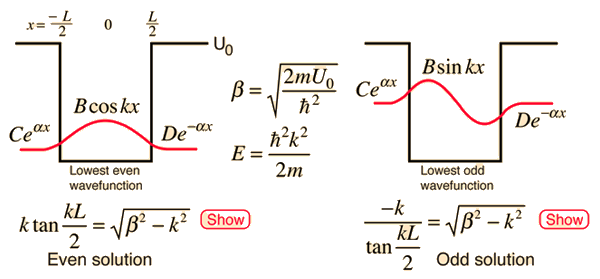Index

Schrodinger equation concepts

 HyperPhysics***** Quantum Physics R Nave
Go Back

# Even Solution, Finite BoxThis last step makes use of the substitution that was used in the setup of the finite well problem: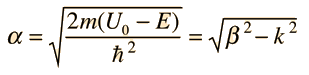The standard constraints on the wavefunction require that both the wavefunction and its derivative be continuous at any boundary. Applying such constraints is often the way that the solution is forced to fit the physical situation.

### Numerical solution for ground state

Index

Schrodinger equation concepts

 HyperPhysics***** Quantum Physics R Nave
Go Back

# Numerical Solution for Ground State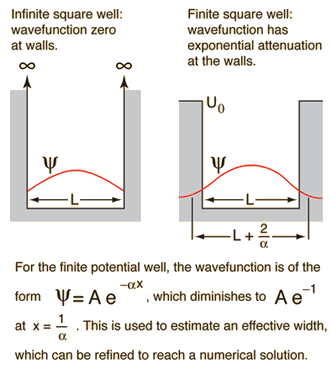The ground state solution for a finite potential well is the lowest even parity state and can be expressed in the form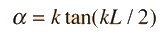where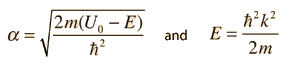. Since both sides of the equation are dependent on the energy E for which you are solving, the equation is trancendental and must be solved numerically. One way to estimate the ground state energy of a finite potential well is to use the infinite well energy to produce a trial attenuation factor α. Then the value of α can be refined by iteration to get an effective well width and a numerical solution for the energy.
For well width L = x 10^ m = nm= fermi,

and mass = x 10^ kg = me = mp = MeV/c2,

the infinite well ground state (n=1) energy is E = x 10^ joule = eV= MeV, = GeV.

For potential U0 = x 10^ joule = eV= MeV,
a first estimate of the attenuation coefficient = x10^m-1 .
This gives a refined effective well width of L = x 10^ m = nm= fermi,
and a refined ground state energy estimate:
E = x 10^ joule = eV= MeV, = GeV.

This calculation process works best when the potential is very large compared to the calculated energy. To evaluate the convergence, the process can be repeated.

 Iteration Effective well width (m) Estimate of energy (eV) 1 x10^ x10^ 2 x10^ x10^ 3 x10^ x10^ 4 x10^ x10^
Index

Schrodinger equation concepts

 HyperPhysics***** Quantum Physics R Nave
Go Back

# Odd Solution, Finite Box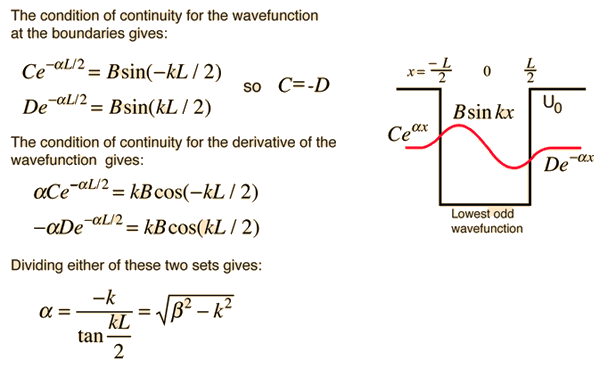This last step makes use of the substitution that was used in the setup of the finite well problem:The standard constraints on the wavefunction require that both the wavefunction and its derivative be continuous at any boundary. Applying such constraints is often the way that the solution is forced to fit the physical situation.

Index

Schrodinger equation concepts

 HyperPhysics***** Quantum Physics R Nave
Go Back

# Parity and the Particle in a Box

In the one-dimensional case, parity refers to the "evenness" or "oddness" of a function with respect to reflection about x=0.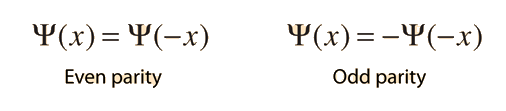The particle in a box problem can give some insight into the importance of parity in quantum mechanics. The box has a line of symmetry down the center of the box (x=0). Basic considerations of symmetry demand that the probability for finding the particle at -x be the same as that at x. The condition on the probability is given by:This condition is satisfied if the parity is even or odd, but not if the wavefuntion is a linear combination of even and odd functions. This can be generalized to the statement that wavefunctions must have a definite parity with respect to symmetry operations in the physical problem.

Index

Schrodinger equation concepts

 HyperPhysics***** Quantum Physics R Nave
Go Back